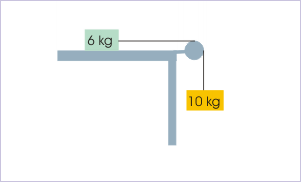# 13.12 Conservation of energy in mechanical process  (Page 2/2)

 Page 2 / 2

$⇒{W}_{E}+{W}_{F}=\Delta K+\Delta U$

We are required to find minimum force. We need to understand the implication of this phrase. It is clear that we can apply external force in such a manner that block of “2 kg” acquires kinetic energy by the time block of “1 kg” is initiated in motion. Alternatively as a base case, we can apply external force gradually and slowly in increasing magnitude till the block of “1 kg” is initiated in motion. In this case, the block of “2 kg” does not acquire kinetic energy. This mode of application of external force represents the situation when minimum external force will be required to initiate block of “1 kg”. Hence,

$\Delta K=0$

Motion of block is constrained in horizontal direction. There is no change of vertical elevation. Hence, there is no change in gravitational potential energy. However, spring is stretched from its neutral state. As a consequence, there is change in elastic potential energy of the spring. Let “x” be the extension in the spring by the time block of “1 kg” is initiated in motion. Then, the total change in potential energy is :

$\Delta U=\frac{1}{2}k{x}^{2}$

The work by friction is done only on the right block of “2 kg” :

${W}_{F}=-\mu {m}_{2}gx$

On the other hand, the work by external force is :

${W}_{E}=Fx$

Putting all these values in the equation of conservation of energy :

$⇒Fx-\mu {m}_{2}gx=\frac{1}{2}k{x}^{2}$

$⇒F-\mu {m}_{2}g=\frac{1}{2}kx$

Clearly, we can not solve this equation as there are two unknowns, “F” and “x”. We, therefore, make use of the fact that spring force on the block of mass “1 kg” is equal to maximum static friction,The spring force is equal to maximum static friction.

$kx=\mu {m}_{1}g$

Combining two equations, we have :

$⇒F-\mu {m}_{2}g=\frac{1}{2}\mu {m}_{1}g$

$⇒F=\mu g\left({m}_{1}+\frac{{m}_{2}}{2}\right)=0.5X10\left(1+\frac{2}{2}\right)X10=10\phantom{\rule{1em}{0ex}}N$

It is interesting to note that force required to initiate left block is independent of spring constant.

## Mechanical process without external force on the system

Since no external force operates on the system, there is no work by external force. The system under consideration is, therefore, an isolated system. The form of conservation law for general mechanical process is further reduced as :

${W}_{F}=\Delta K+\Delta U$

In words, we can put the conservation of energy for mechanical process under given condition as :

“Work by friction within an isolated system is equal to the change in potential and kinetic energy of the system.”

## Example

Problem 2: In the arrangement shown, the block of mass 10 kg descends through a height of 1 m after being released. The coefficient of friction between block and the horizontal table is 0.3, whereas pulley is friction-less. Considering string and pulley to be “mass-less”, find the speed of the blocks.Blocks move by 1 m.

Solution : Here, friction is present as internal force to the system. Hence, we use the form of conservation law as :

${W}_{F}=\Delta K+\Delta U$

Let us denote 6 kg and 10 kg blocks with subscript “1” and “2”. There is no change of height for the block on the table. Only change in gravitational potential energy is due to change in the height of the block hanging on the other end of the string. Thus, change in potential energy is :

$\Delta U={m}_{1}gh+{m}_{2}gh=0-10x10x1=-100\phantom{\rule{1em}{0ex}}J$

Two blocks are constrained by a taut string. It means that both blocks move with same speed. Let the speed of the blocks after traveling “1 m” be "v". Now, initial kinetic energy of the system is zero. Therefore, the change in kinetic energy is given by :

$\Delta K=\frac{1}{2}{m}_{1}{v}^{2}+\frac{1}{2}{m}_{2}{v}^{2}$

$⇒\Delta K=\frac{1}{2}X6X{v}^{2}+\frac{1}{2}X10X{v}^{2}$

$⇒\Delta K=8{v}^{2}$

Friction works only on the block lying on the table. Here, work by friction is given as :

${W}_{F}=-\mu Nx=-\mu {m}_{1}gx=-0.3X6X10X1=-18\phantom{\rule{1em}{0ex}}J$

Putting in the equation of conservation of energy,

$⇒-18=8{v}^{2}-100$

$⇒{v}^{2}=\frac{82}{8}$

$⇒v=3.2\phantom{\rule{1em}{0ex}}m/s$

#### Questions & Answers

What are the system of units
Jonah Reply
A stone propelled from a catapult with a speed of 50ms-1 attains a height of 100m. Calculate the time of flight, calculate the angle of projection, calculate the range attained
Samson Reply
58asagravitasnal firce
Amar
water boil at 100 and why
isaac Reply
what is upper limit of speed
Riya Reply
what temperature is 0 k
Riya
0k is the lower limit of the themordynamic scale which is equalt to -273 In celcius scale
Mustapha
How MKS system is the subset of SI system?
Clash Reply
which colour has the shortest wavelength in the white light spectrum
Mustapha Reply
how do we add
Jennifer Reply
if x=a-b, a=5.8cm b=3.22 cm find percentage error in x
Abhyanshu Reply
x=5.8-3.22 x=2.58
sajjad
what is the definition of resolution of forces
Atinuke Reply
what is energy?
James Reply
Ability of doing work is called energy energy neither be create nor destryoed but change in one form to an other form
Abdul
motion
Mustapha
highlights of atomic physics
Benjamin
can anyone tell who founded equations of motion !?
Ztechy Reply
n=a+b/T² find the linear express
Donsmart Reply
أوك
عباس
Quiklyyy
Sultan Reply
Moment of inertia of a bar in terms of perpendicular axis theorem
Sultan Reply
How should i know when to add/subtract the velocities and when to use the Pythagoras theorem?
Yara Reply

### Read also:

#### Get the best Physics for k-12 course in your pocket!

Source:  OpenStax, Physics for k-12. OpenStax CNX. Sep 07, 2009 Download for free at http://cnx.org/content/col10322/1.175
Google Play and the Google Play logo are trademarks of Google Inc.

Notification Switch

Would you like to follow the 'Physics for k-12' conversation and receive update notifications?ByBy David Martin